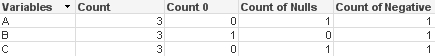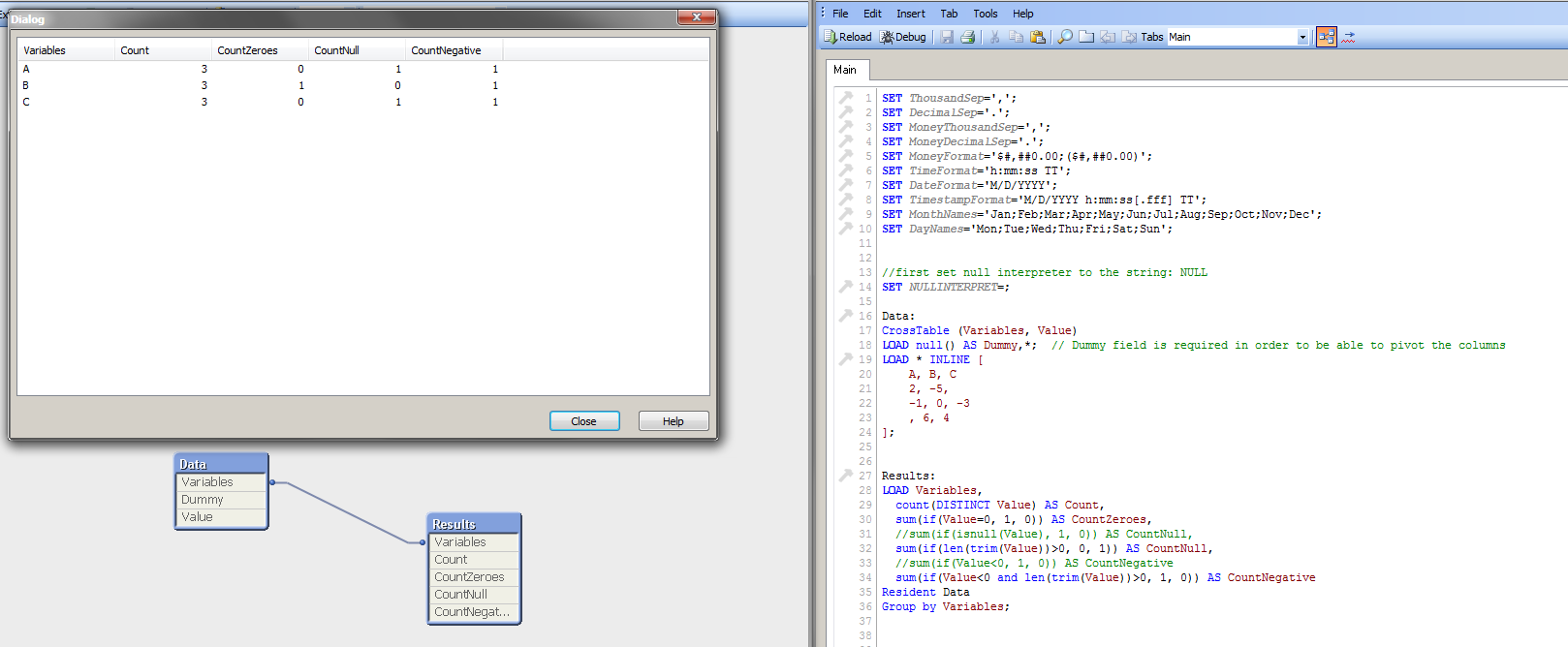# QlikView App Dev

Discussion Board for collaboration related to QlikView App Development.

Announcements
On-Demand Webinar: Learn proven best practices for a smooth transition to Qlik Sense® SaaS. REGISTER
cancel
Showing results for
Did you mean:Not applicable

## Table of statistics in QV

Hi,

I'm a new user to QV. Not sure how to solve the following issue, any experts can help?

I have a dataset in excel file as follows:

ABC
2-5
-10-3
64

I want to import this file in QV and generate the following statistical table as a result:

VariablesCountCount of ZerosCount of NullsCount of negative values
A3001
B3101
C3011

Anyone know how to do it? Thanks!

12 RepliesPartner - Creator III

Hi you can either achieve this by script or in the layout, Here is a solution in script :

Data:

CrossTable (Variables, Value)

LOAD null() AS Dummy, *;  // Dummy field is required in order to be able to pivot the columns

LOAD * INLINE [

A, B, C

2, -5

-1, 0, -3

, 6, 4

];

Results:

count(DISTINCT Value) AS Count,

sum(if(Value=0, 1, 0)) AS CountZeroes,

sum(if(isnull(Value), 1, 0)) AS CountNull,

sum(if(Value<0, 1, 0)) AS CountNegative

Resident Data

Group by Variables;

Hope it helps,

MichaelMVP

and here in the layout (chart)

see attachmentNot applicable
Author

Thanks Michael! It really helps!Not applicable
Author

Thanks Massimo! I created the qvw file using your template, it works well.

Another thing I want to ask is, how to add a list box of a condition there? For example, what shall I do if I want to see how the counts change where A is not null.

Traditionally, I would add a list box and select variable A and make the restriction on it, but since you already created the cross table, I cannot select variable A anymore...I only three variables now: variables, value and dummy. Do you know how to solve this?

Many thanks!!!!!Not applicable
Author

Hi,

Easiest way to flag nulls is during the initial load; for example:

LOAD A, B, C, if(Len(A)=0, 'Yes', 'No') as [A is null]

FROM (Excel source)

;

I used Len()=0 to identify the empty cells; you also have Isnull() function, but Len()=0 also works on empty strings.

Then you can use [A is null] as filter, and check the change in your pivot chart.

Hope this helpsNot applicable
Author

Hi Pierre, thanks for your suggestion! As Michael suggested, I used the following script to load the data, so it will create a cross table for me. However, if I do so, I will only return three variables: "Variables", "Value" and "Dummy", and I was not able to select any of my original variables such as A, B, C. Do you know where I should insert your "if(Len(A)=0, 'Yes', 'No') as [A is null]" into my existing script?

Thank you in advance!

My existing script:

Data:

CrossTable (Variables, Value)

LOAD null() AS Dummy, *;  // Dummy field is required in order to be able to pivot the columns

LOAD * INLINE [

A, B, C

2, -5

-1, 0, -3

, 6, 4

];Not applicable
Author

OK. Then you need to add the flags before turning it into a crosstable.

Data:

CrossTable (Variables, Value)

LOAD null() AS Dummy, *;  // Dummy field is required in order to be able to pivot the columns

LOAD *, if(Len(A)=0, 'Yes', 'No') as [A is null];

LOAD * INLINE [

A, B, C

2, -5

-1, 0, -3

, 6, 4

];

If you make Results table in script, you need to add new results in script too:

Results:

count(DISTINCT Value) AS Count,

sum(if(Value=0, 1, 0)) AS CountZeroes,

sum(if(isnull(Value), 1, 0)) AS CountNull,

sum(if(Value<0, 1, 0)) AS CountNegative,

count(DISTINCT if([A is null]='No', Value)) AS CountANotNull,

sum(if(Value=0 and [A is null]='No', 1, 0)) AS CountZeroesANotNull,

sum(if(isnull(Value) and [A is null]='No', 1, 0)) AS CountNullANotNull,

sum(if(Value<0 and [A is null]='No', 1, 0)) AS CountNegativeANotNull

Resident Data

Group by Variables;

As you see, it's getting a bit heavy. Making Results in layout allows to use a simple filter, so it's more flexible at this point.Not applicable
Author

Hi Pierre, I tried your new script by adding this [A is null] before the load of A,B,C, but it doesn't work...My problem is that when creating a cross table, I'm not able to add a list box of the original variable A. My goal is to realize the dynamic changing of the cross table when my condition for A changes.

See the pics attached. After I create the cross table, I can only select "Dummy", "Variables", "Value" in <Field>.For the Result table, I prefer to do it in the layout instead of script. So don't worry about this part for now. Is it possible for you to attach me the .qvw file if you know how to solve the above problem?

Thanks a lot for your help and patience!Creator III

Hey Michael,

I had to modify the script to below in order to get the Variable Table to show the proper results for me.

//first set null interpreter to the string: NULL

SET NULLINTERPRET=;

Data:

CrossTable (Variables, Value)

LOAD null() AS Dummy,*;  // Dummy field is required in order to be able to pivot the columns

LOAD * INLINE [

A, B, C

2, -5,

-1, 0, -3

, 6, 4

];

Results:

count(DISTINCT Value) AS Count,

sum(if(Value=0, 1, 0)) AS CountZeroes,

//sum(if(isnull(Value), 1, 0)) AS CountNull,

sum(if(len(trim(Value))>0, 0, 1)) AS CountNull,

//sum(if(Value<0, 1, 0)) AS CountNegative

sum(if(Value<0 and len(trim(Value))>0, 1, 0)) AS CountNegative

Resident Data

Group by Variables;If i use the script the way you provided (snapshot below) I get the wrong results.  I believe it is the way the Null values are being handled in the Inline Load.  If someone could explain why in further detail I would much appreciate it.